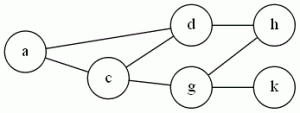# Vertex Cover

Back to the “core 6” with a classic problem from graph theory.  There are lots of similar graph problems, so it’s important to keep them all straight.

The Problem: Vertex Cover (VC)

The Definition: Given a graph G=(V,E) and a positive integer K (G&J say that K ≤ |V|, but really, if it’s bigger than that, you can have the algorithm just return “yes”).  Can I find a subset V’ of V, |V’| ≤ K, where every edge in E has at least one endpoint in V’?

Example: Hmm, I wonder if I can insert an image..Ok, looks like that worked.  In the graph above, {c,d,g} is a vertex cover, because all edges have at least one endpoint in the set {c,d,g}.  As we’ll see later, a graph containing a clique (complete subgraph) of N nodes will need at have at least N-1 of those vertices in the cover.

The reduction: From 3SAT.  G&J on pages 54-56 show the construction.  We build a graph with vertices for all variables and their negation, with an edge between them.  We have three variables for each clause, connected in a triangle.  (The three variables correspond to “positions” in the clause).  Then there is an edge from each vertex corresponding to a literal (or its negation) to its corresponding “clause vertex”- the vertex that holds the position of that variable in the original formula.  K= # of variables + 2* number of clauses.

Difficulty: 7 , assuming it’s the first time a student has seen this sort of reduction.  If they have seen something similar (for example, a reduction from 3SAT to Clique), then maybe a 5.  The ideas are pretty similar.

Note: I teach my algorithms class using the Cormen book.  In it, they reduce 3SAT to Clique, proving Clique is NP-Complete, and then reduce Clique to VC. This works in the exact same way as the reduction from VC to Clique that I’ll be doing here next.